## 音箱分频器在线计算公式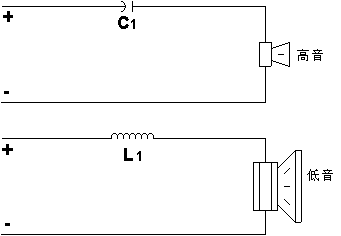C1= μf

L1= mH

 . 相移的一阶分频器是90度

: 欧姆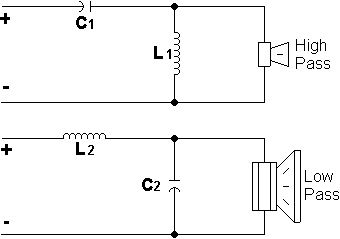C1 = μf

L1 = mH

C2 = μf

L2 = mH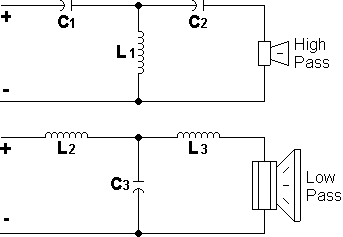C1 = μf
C2 = μf
L1 = mH

L2 = mH
L3 = mH
C3 = μf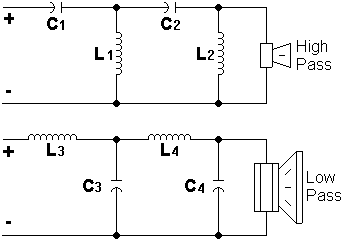C1 = μf
C2 = μf
L1 = mH
L2 = mH

C3 = μf
C4 = μf
L3 = mH
L4 = mH

Zobel瑟贝尔电路（阻抗稳定）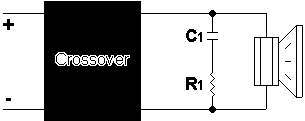C1= μf

R1= 欧姆

L型扬声器喇叭衰减电路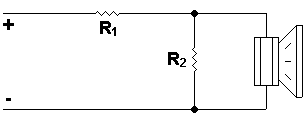R1 = 欧姆

R2 = 欧姆